# Galaxy project

### By: Han de Bruijn Jan 2016 / Nov 2019

$\def \hieruit {\quad \Longrightarrow \quad} \def \slechts {\quad \Longleftrightarrow \quad} \def \SP {\quad ; \quad} \def \MET {\quad \mbox{with} \quad}$ A standard book about astronomy is:
THE PHYSICAL UNIVERSE
An Introduction to Astronomy

by Frank H. Shu

And, according to that standard book, there is the Missing Mass Problem, as explained here: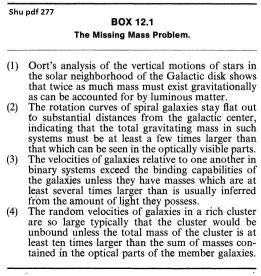In my humble opinion - I'm not an astronomer - it's a bit suspect that anything in the distant cosmos appears to be oversized. And the more distant, the more oversized. It's not impossible that flaws in the Cosmic Distance Ladder (Wikipedia) are to be hold responsible for this, as is argued in Incorrect Assumptions in Astrophysics. While this might provide an explanation for the issues (1), (3) and (4) in Box 12.1, it definitely does not clarify issue (2): the flat Galaxy rotation curves:At an alternative website, called The 100 Year Wrong Turn in Cosmology, we find a PDF document with the intriguing title "Galactic mass distribution without dark matter or modified Newtonian mechanics". It is written by Kenneth F Nicholson, a retired engineer. A more elaborate paper with analogous content is the PDF titled "Mass Distribution in Rotating Thin-Disk Galaxies According to Newtonian Dynamics", by James Q. Feng and C. F. Gallo. A very much improved version of the latter paper is:

### Deficient Reasoning for Dark Matter in Galaxies

Could this be possible? That part of the Missing Mass Problem can be solved by simple and straightforward application of classical Newtonian mechanics? And that part of the problem has come into existence solely by erroneous calculation? Seems unbelievable! But anyway, it's quite worthwhile to check it all out. Now!

According to Newton's law of universal gravitation, the attractive force ($F$) between two bodies is directly proportional to the product of their masses ($m_1$ and $m_2$), and inversely proportional to the square of the distance ($r$ : inverse-square law) between them (see Wikipedia): $$F = G\frac{m_1 m_2}{r^2}$$ The constant of proportionality ($G$) is the gravitational constant: $$G = 6.674\,08(31) \times 10^{-11}\,m^3\,kg^{-1}\,s^{-2}$$ Newton's law of gravitation shall be applied to a RingWorld of gravitating matter, to begin with.

## RingWorld Integral

Consider a ring (= circle) with a uniform mass density distribution. The RingWorld has a center $M$ and a radius $R$: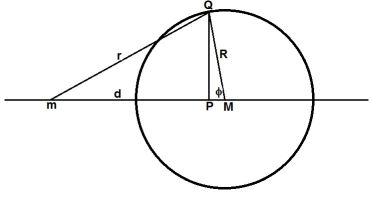Let $m$ be a test mass at some distance $d$ from the center $M$ of the RingWorld. Let $Q$ be the position of an infinitesimal element in the Ring. The mass $m_1$ in Newton's law shall be identified with the test mass $m$. Let the mass density (i.e. mass per unit length) in the ring be equal to $\rho$. Then the infinitesimal element at $Q$ has a mass $\rho\,R\,d\phi$ , to be identified with $m_2$. So Newton's law becomes, for the gravitational attraction between the test mass and an infinitesimal part of the ring: $$dF = G\frac{m \times \rho\,R\,d\phi}{r^2}$$ Where $r$ is the distance between the test mass $m$ and the infinitesimal element at $Q$. Due to symmetry, straight lines through the center of the ring must be all equivalent. For simplicity, select the line through the x-axis for measuring forces. Polar coordinates are to be employed. Then, according to Pythagoras' theorem and some simple trigonometry: $$r^2 = (\overline{Mm}-\overline{MP})^2+(\overline{PQ})^2 = \left[d-R\cos(\phi)\right]^2+\left[R\sin(\phi)\right]^2 \quad \Longrightarrow \\ r^2 = d^2-2Rd\cos(\phi)+R^2$$ Due to symmetry, we only need to consider the component of the force that is directed from $m$ to $M$, being a projection: $$\overline{Pm}/\overline{Qm} = \frac{d-R\cos(\phi)}{r}$$ Herewith Newton's law becomes, with $r^3$ in the denominator: $$dF = G\frac{m\times\rho\,R\,d\phi}{r^2} \times \frac{d-R\cos(\phi)}{r} = m \times G\frac{\rho\,R\,\left[d-R\cos(\phi)\right]\,d\phi}{\left[d^2-2Rd\cos(\phi)+R^2\right]^{3/2}}$$ The total attractive force at the test mass, exerted by the ring, is the integral of this. Due to symmetry, half of the computational effort can be saved. $$F = m \times 2 \int_0^\pi G\frac{\rho\,R\,\left[d-R\cos(\phi)\right]\,d\phi}{\left[d^2-2Rd\cos(\phi)+R^2\right]^{3/2}}$$ Make the result dimensionless as much as possible: $$F(d/R) = m \times G \rho/R \times 2 \int_0^\pi \frac{\left[(d/R)-\cos(\phi)\right]\,d\phi}{\left[(d/R)^2-2(d/R)\cos(\phi)+1\right]^{3/2}}$$ This formula is valid even for test masses inside the ring: not only for $d > R$ but also for $d < R$. The function $F(d/R)$ is depicted in the figure below. Function value positive means that the force vector is directed from the left to the right. Function value negative means that the force vector is directed from the right to the left.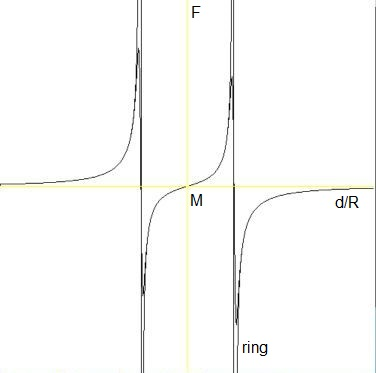A special case is a test mass at the ring. Then the integral becomes, for $d/R=1$: $$F = m \times G \rho/R \times 2 \int_0^\pi \frac{\left[1-\cos(\phi)\right]\,d\phi}{\left[1-2\cos(\phi)+1\right]^{3/2}} = m \times G \rho/R \times \frac{1}{\sqrt{2}} \int_0^\pi \frac{d\phi}{\sqrt{1-\cos(\phi)}}$$ Quite unfortunately, this special integral is singular; it has an infinite outcome, because the denominator of the integrand is zero for $\phi=0$. How do we deal with that?
The answer is that we don't! Take a look at the above graph. If the test mass $m$ is close to the ring, then it will experience a strong force towards the ring. This simply means that a test mass cannot escape from its RingWorld. And that's all. A test mass in the ring does not contribute to anything else than just staying there. Therefore what we shall do is: simply skip / ignore the infinite contribution.

## Mathematical model

Mathematics is not reality. Mathematics is fantasy. But it is tamed fantasy. Once you have established the basic hypotheses, there is no way out. You have to follow the logic and accept the consequences, whatever they are right or wrong. So what we shall do now is to make a mathematical model of a galaxy, knowing that it is of limited validity when compared with its immense reality. It will be assumed that the galaxy can be modeled as a flat disk. The flat disk, in turn, is thought as being built up from (in)finitely thin RingWorlds: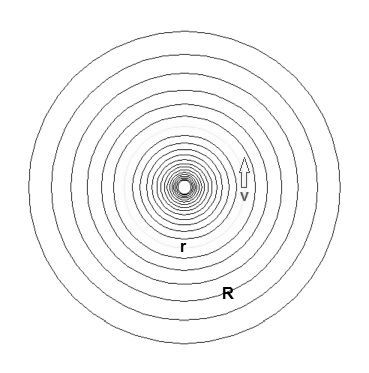Employing the end result from the preceding section, we first replace $d$ by $r$ - what's in a name, huh? Then we have, for $\;r/R\ne 1$ and assuming that $\rho(R)$ (the density of the stars) is a function of the radius only: $$F_\mbox{ring}(r/R) = m \times G\,\rho(R)/R \times 2 \int_0^\pi \frac{\left[(r/R)-\cos(\phi)\right]\,d\phi}{\left[(r/R)^2-2(r/R)\cos(\phi)+1\right]^{3/2}}$$ This result must be integrated (i.e summed) over all rings with radius $R$. Since we are only interested in relative outcomes, apart from scaling, the range of $R$ shall be conveniently limited to the interval $R \in \left[0,1\right]$. $$F_\mbox{galaxy}(r) = \int_0^1 F_\mbox{ring}(r/R) dR = m \times G \int_0^1 \left[ 2 \int_0^\pi \frac{\left[(r/R)-\cos(\phi)\right]\,d\phi}{\left[(r/R)^2-2(r/R)\cos(\phi)+1\right]^{3/2}} \right] \rho(R)/R\; dR$$ This is the total force exerted by all rings in the galaxy on a test particle in the galaxy. The test particle is part of the ring with radius $r$. That ring is assumed to rotate with uniform velocity $v$, thus creating a centripetal force which is opposite and equal to the gravitational force of the galaxy. The expression for this centripetal force is well known. Thus we have: $$F = \frac{m\,v^2}{r} = F_\mbox{galaxy}(r)$$ Equating the two shows that the result is independent of the test mass $m$ : $$v(r)^2 = G \int_0^1 \left[ 2 \int_0^\pi \frac{\left[(r/R)-\cos(\phi)\right]\,d\phi}{\left[(r/R)^2-2(r/R)\cos(\phi)+1\right]^{3/2}} \right] (r/R)\,\rho(R)\;dR$$ There is one important condition, though: $v^2 > 0$ . So the right hand side must be positive. Otherwise it would be impossible for the RingWorld to compensate gravity by means of a centripetal force. Herewith one of the serious limitations of the mathematical model has become obvious already. Furthermore we can see that the gravitational constant $G$, being only a (positive) scaling factor, can be dropped for our purpose - or merged with the speed as $v/\sqrt{G}$. While it is noted that the gravitational force can be wildly negative close to the center of the galaxy, one thing is for sure: $F_\mbox{galaxy}(r=0) = 0$, so there is no counter force needed exactly in the center.

## Results

It's virtually impossible to calculate the Galaxy Integral analytically. Therefore an application has been developed - formerly called "computer program", nowadays called "app". It's written in the (Delphi) Pascal programming language. The app shall be in the public domain and it is accompanied by CopyLefted
• ### Source Code

A significant detail is: how to design a proper computational grid? I have cooked up two flavors of them:
• Arithmetical grid.
In radial direction: $R = (i+1)/(N+1)$ with $i = 0,1, \cdots ,N$.
In angular direction: $\phi = j\cdot 2\pi/M$ with $j = 0,1 \cdots , M-1$.
• Geometrical grid.
Much more complicated, as is explained in a separate webpage.
Both grids have been refined a couple of (4) times, doubled to be precise: $N := 2\times N \; ; \; M := 2\times M$. The more refined the grid, the more black (less grey) the accompanying graph of the star speed profile $v(r)$. It's a nasty habit of mine not to present numerical results any more fancy than they actually are. Thus to emphasize once more that a mathematical model is not reality. It may be an advantage, for example, that you can still observe some of the layout of courser grids (in the grey curves). Together with the fact that the two grids give slightly different outcomes.

Crucial for success of the calculations is the (observed) mass density profile of the stars in the galaxy. For a novice in the field, it's not easy to find something sensible in the references available on the internet. After some search I've decided to take this one, on page 19. In the figure below, the graph of this function is sketched in $\color{red}{red}$. There is another scaling factor involved that norms the mass of the galaxy to unity, according to $\int_0^1 \rho(R)\cdot2\pi R\,dR = 1$. Apart from such minor details, this is what we finally have in the program: $$\rho(R) \sim \left[1+(R/\sigma)^2\right]^{-3/2} \quad \mbox{where} \quad 0 \le R \le 1 \quad \mbox{and} \quad \sigma \approx 0.1$$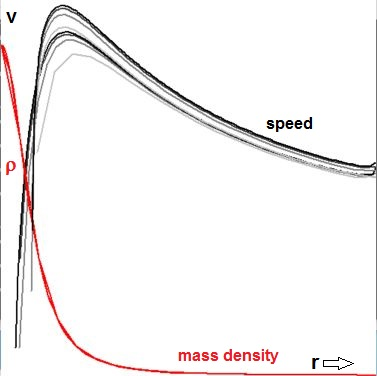I think that the conclusion is clear. If the adopted mass density profile of the stars is indeed acceptable, then the galaxy rotation speed profile $v(r)$ is not according to the observations. This means that I've not yet ( 2016 ) been able to reproduce the claims in the papers by Kenneth F Nicholson and James Q. Feng and C. F. Gallo.
Note. These papers are deviant from the webpage you are reading in that they handle an inverse problem: how to determine the mass density distribution from a given velocity profile.

So there is no reason to believe in a Deficient Reasoning for Dark Matter in Galaxies as far as we can see. Does that mean that evidence for dark matter in galaxies is substantial instead? Yes and no! As we shall shortly see, there is an alternative Basic Hypothesis. It sounds like: The rest mass of atomic particles is increasing with (proper) time. If this is true, then dark matter apart from common matter may not be much of an issue. Varying elementary particle rest mass can be conceived as dark mass in diguise. Dark matter is not outside but it rests inside common matter.

## Theory for Halton Arp

The basic ideas of the Theory have been formulated by Halton Arp, mainly in common speech. Responsibility for the mathematics of Halton Arp's Theory, as formulated on this website, is mine (Han de Bruijn). Prerequisite reading:

### Basic HypothesisIntrinsic RedshiftLength ContractionTime DilationNarlikar's Law

Once we have gathered this knowledge, the basics of the calculations in this webpage may be reconsidered properly. Actually it's nothing else than the laws of motion by Newton, resulting in a - albeit rather complicated - modification of the following: $$F = m.a = m \frac{v^2}{r} = G\sum_k \frac{m\,M_k}{R_k^2} \MET v = \frac{ds}{dT}$$ Quantities: $F=$ force, $m=$ test mass, $a=$ acceleration, $v=$ speed, $r$ = distance to galaxy center, $G=$ gravitational constant, $M_k=$ stellar masses, $R_k=$ distance between $m$ and $M_k$, $ds=$ infinitesimal increment in space, $dT=$ infinitesimal increment in orbital time. The latter emphasis is important, as we shall shortly see.
Assume for the moment that all size within the galaxy is scaled as $r'=r/L$ , $R_k'=R_k/L$, all mass as $M_k'=M_k/W$, the velocity of the test mass as $v'= v/V$, where $L=$ radius of the galaxy, $W=$ total mass of the galaxy, $V=$ some characteristic velocity. Then we have, with a technique known as Nondimensionalization: $$\frac{v'^2}{r'}\frac{V^2}{L} = G\frac{W}{L^2}\sum_k \frac{M_k'}{R_k'^2} \hieruit \frac{v'^2}{r'}\frac{V^2.L}{G.W} = \sum_k \frac{M_k'}{R_k'^2} \hieruit v' = \sqrt{\frac{G.W}{V^2.L}}\sqrt{r'\sum_k \frac{M_k'}{R_k'^2}}$$ Which is more or less the - dimensionless - result as employed in our calculations. But we want to know for sure that the factor $\sqrt{G.W/(v^2.L)}$ is just dimensionless scaling: $$[G] = m^3kg^{-1}s^{-2} \SP [W] = kg \SP [V] = m\,s^{-1} \SP [L] = m \\ \hieruit \frac{[G][W]}{[V^2][L]} = \frac{m^3kg^{-1}s^{-2}.kg}{(m\,s^{-1})^2m} = 1$$ Empty space and mass are the only things that are involved with the right hand side. And this is what we know from Time Dilation:
• With Newton's laws alone - that is: with inertia and gravity and nothing else - it makes no difference that elementary particle rest mass is varying.
But the left hand side is different: space and time are involved with $v'$. Empty space is insensitive to varying elementary particle rest mass, but we have seen that such is not the case with time. Let's replace the primed variables in our formulas by common ones for ease of further discussion. It is clear then that the velocity $v$ is the derivative of a distance $s$ in empty space to time in Newtonian mechanics, which then must be orbital time $T$. This is not how time is observed in astronomy, though. Spectral data are abundant in astronomy, that's one reason why. Moreover, according to modern definitions for space and time measurement, time is measured as atomic time. So let the velocity $u$ in atomic time $t$ be the desired end-result, then without Halton Arp's Theory nothing will happen. But if varying elementary particle rest mass is implemented, then, according to the laws of Time Dilation: $$u = \frac{ds}{dt} = \frac{ds}{dT}\frac{dT}{dt} = v \frac{\sqrt{m_0/m}}{m_0/m} = v\sqrt{m/m_0}$$ Mass and clocks as present at some fixed here and now are associated with elementary particle rest mass $m_0$. Remember that all of our calculations are dimensionless, meaning that only proportions do really count. As a consequence, if elementary particle rest mass is varying across the galaxy, then the above correction should be applied to the calculated velocities, in order to represent the observed velocities. Let us assume that elementary particle rest mass is indeed varying across the galaxy, in such a way that new mass is created in the center and becomes more aged in outward direction. From the section about Intrinsic Redshift it can be inferred that the elementary particle rest mass in galaxies can be compared with some reference elementary particle rest mass $m_0$ with help of a Length Contraction law: $$\frac{m_0}{m} = \frac{\lambda}{\lambda_0} = 1+z \hieruit u = v\sqrt{\frac{\lambda_0}{\lambda}} = v\sqrt{1/(1+z)}$$ So, if we were able to measure the redshift across a galaxy, then it should be possible to come up with a precise profile of changing elementary particle rest mass, as a function of the distance $r$ to the center. At this moment, not being an astronomer myself, I do not have such redshift data at my disposal - provided that these data do indeed exist. The only thing that we can show now is a beautiful picture of a galaxy. Is it suggesting something of the kind?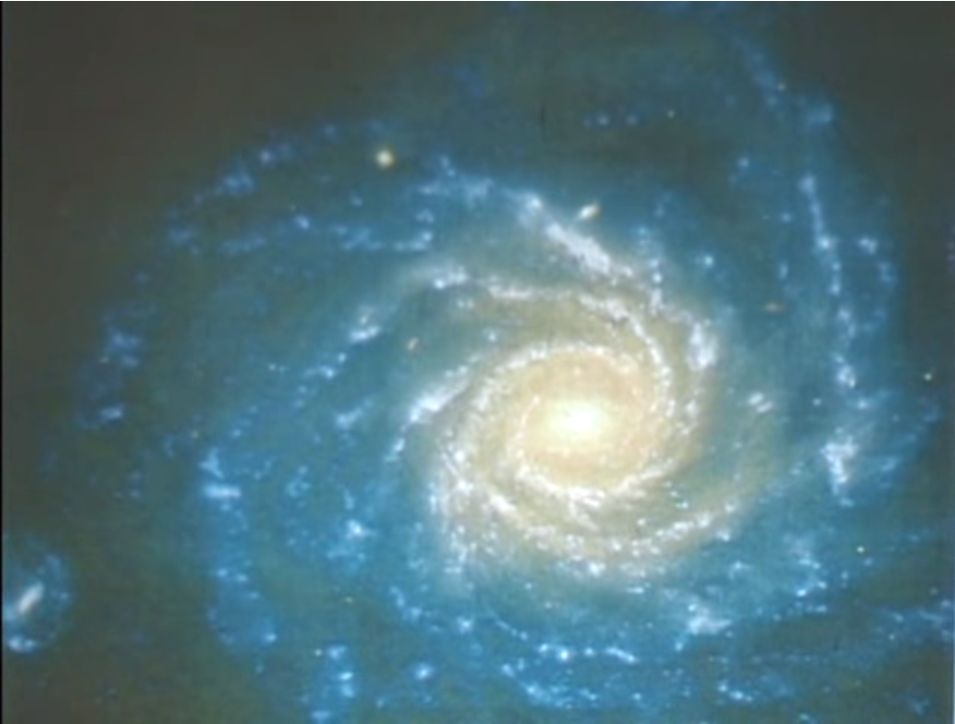So let's make a wild guess for the moment being: elementary particle rest mass is proportional to the distance from the center of the galaxy. Formally: $m \sim r$. Giving, in common speech: $$\mbox{correct rotation curve} = \sqrt{\mbox{distance to nucleus}} \times \mbox{wrong rotation curve}$$ This certainly is a daring hypothesis, but if we implement this in our app, then we get the following result, picture on the left: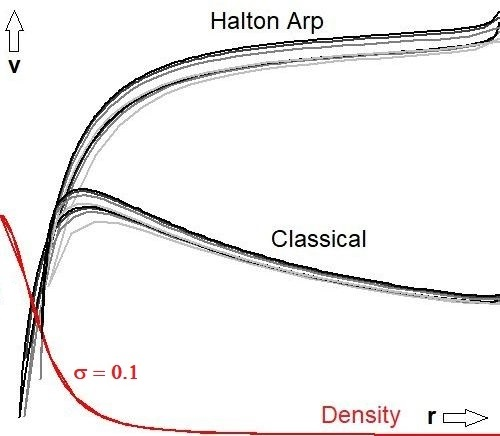The modified app shall be in the public domain as well and it is accompanied too by Source Code; search for "Halton Arp" in the source.

## Empty Hole Photography

In a recent (2019 April 10) article, titled First M87 Event Horizon Telescope Results, it is claimed that The Event Horizon Telescope (EHT) has mapped the central compact radio source of the elliptical galaxy M87 at 1.3 mm with unprecedented angular resolution. The accompanying "photograph of a black hole", as I've seen it for the first time, can be found at the Sterrenstof forum.
Despite all this effort by established science, I'm sorry to say that quite another picture is emerging with Halton Arp's Theory. Only a brief sketch of this Alternative Cosmology shall be given at this website; the reference about Narlikar's Law being a prerequisite reading. The most important point to be made here is supported by the article A Different View of the Universe (Answers in Genesis). Quote mining is in red:
There are NO massive black holes in the center of galaxies. Rather the idea is that new matter emerges into our universe in active galactic nuclei, where Arp suggests there may be white holes rather than black holes. White holes? In fact, there may be nothing there at all. Maybe the center of a galaxy is a place where Matter is created from Nothing; it's an Empty Hole.
Suppose that the center of a galaxy is an Empty Hole indeed. A numerical simulation may be developed in an attempt to reproduce that famous "Black Hole" photograph, but from a completely different theoretical perspective: a ring of newly born stars around an Empty Hole. The end-result is shown below, preceded by the original from First M87 Event Horizon Telescope Results: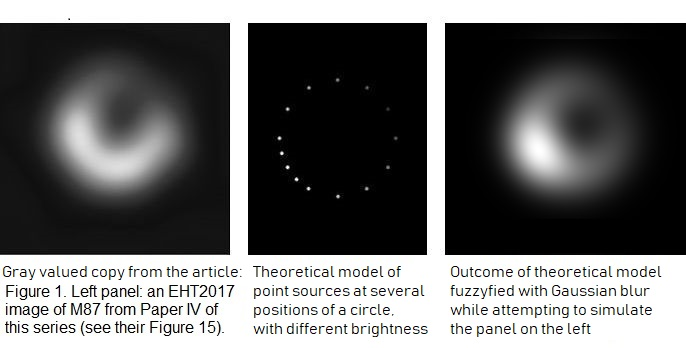Further theoretical underpinning reveals that these sort of "photographs" as such do not contain enough information to make any decisive conclusions possible. This not to say that Halton Arp's white holes are right and Heino Falcke's supermassive black holes are wrong. We have only shown that there is evidence too for the alternative as presented here.